# Linear Momentum, Angular Momentum and Kinetic Energy of Rigid Bodies (Part - 3) Civil Engineering (CE) Notes | EduRev

## Civil Engineering (CE) : Linear Momentum, Angular Momentum and Kinetic Energy of Rigid Bodies (Part - 3) Civil Engineering (CE) Notes | EduRev

The document Linear Momentum, Angular Momentum and Kinetic Energy of Rigid Bodies (Part - 3) Civil Engineering (CE) Notes | EduRev is a part of the Civil Engineering (CE) Course Introduction to Dynamics and Vibrations- Notes, Videos, MCQs.
All you need of Civil Engineering (CE) at this link: Civil Engineering (CE)

The Parallel Axis Theorem

In all the previous calculations we have been calculating the mass moment of inertia about the center of mass. This is what always appears in the angular momentum formula.   But we sometimes want to find the mass moment of inertia about a different point (not the COM), for reasons that will soon be clear.

The mass moment of inertia about an arbitrary point is defined exactly the same way as the inertia about the COM, except that we use the distances from our arbitrary point instead of the distance from the COM.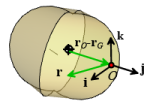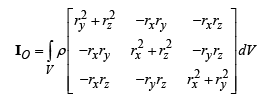It’s painful to have to re-do all these integrals, however.   If we already know IG , the parallel axis theorem lets us calculate IO directly.  Define the vector d that points from G to O

d =rO − rG

Then for a 3D object with mass M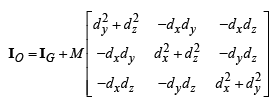For 2D we have a simpler result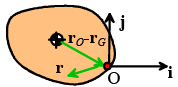IOzz = IGzz + M (dx2 + dy2)

Example: Let’s find the mass moment of inertia of a cylinder about axes that pass through one end of the cylinder (O), instead of the COM.

Here, d=L/2 k⇒dx = dy = 0 dz=L/2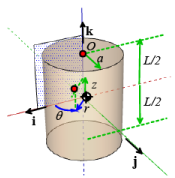The formula gives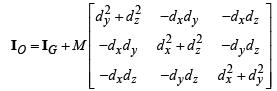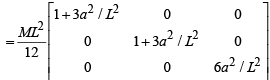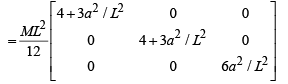6.4.7 Calculating moments of inertia of complex shapes by summation

The most important application of the parallel axis theorem is in calculating the mass moment of inertia of complicated objects (which don’t appear in our table) by adding together moments of inertia for simple shapes. We can illustrate this with a couple of simple examples.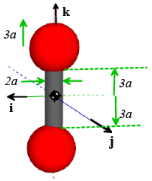Example 1: Two spheres with radius 3a are connected by a rigid cylinder with length 6a and radius a to create a dumbbell. All objects have the same mass density ρ .   Calculate the total mass moment of inertia of the dumbbell.

The general approach is

1. Find the COM of the entire assembly
2. Find the mass moment of inertia of each shape (the spheres and the cylinder) about its own COM
3. Use the parallel axis theorem to find the moment of inertia of each shape about the combined COM
4. Add all the moments of inertia

For our problem

(1)  We know the COM is at the origin by symmetry, so we don’t need to calculate it

(2) The inertia matrices of each object (cylinder + sphere) about their own COM are: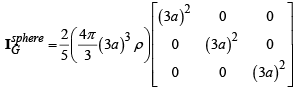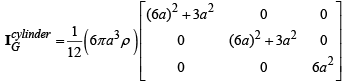(3) We don’t need to use the parallel axis theorem for the cylinder, because its COM is already at the same place as the COM of the assembly. For the spheres, we need to move the COM a distance 6a parallel to the k direction. This means that dx =dy = 0, d z = 6a in our formula.  Therefore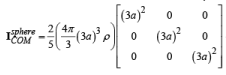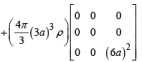(4) We can add everything up (note that there are two spheres).  Its best to use Mupad. The answer is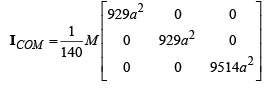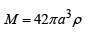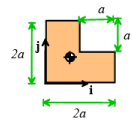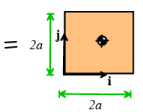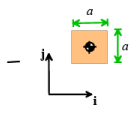Example 2: Things are a lot simpler in 2D. The procedure is the same, but we only need to calculate I zz . For example, to calculate the mass moment of inertia for a square 2ax2a plate with a hole with an axa square cut out from the top corner we would use the following approach.

Start by calculating the total mass and the position of the COM.  We can regard the cut-out section as a square with negative density inside a larger 2ax2a square.

The total mass is therefore M = ρ(2a)2 − ρa2=3ρa2

The position of the COM is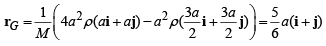The mass moment of inertia of the 2ax2a square and the axa square are

Large square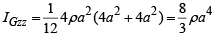(COM at α(i + j ))

Small square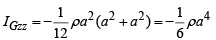(COM at 3/2 α(i + j ) )

We now use the parallel axis theorem to find the moment of inertia of each square about the combined COM.   For the large square: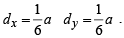For the small square,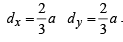The total mass moment of inertia is therefore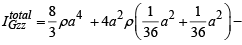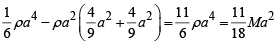6.4.8 Rotating the inertia tensor

All the curious properties of spinning objects – a gyroscope; a boomerang; the rattleback – are consequences of the fact that the mass moment of inertia of an object changes when it is rotated.  We can see this very easily by re-visiting our assembly of masses.  In the original calculation, the red, green and blue masses were located on the i,j,k axes.  We calculated the inertia tensor to be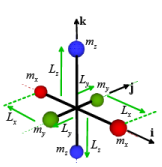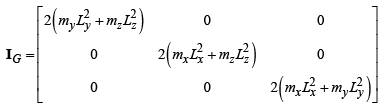Now suppose we rotate the assembly through 90 degrees about the k axis. The red masses now lie on the j axis, and the green ones line up with the i axis.   It is not hard to see that the new mass moment of inertia is now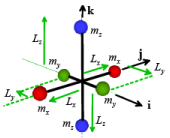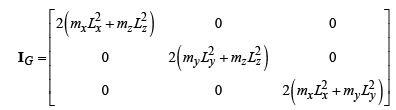( I xx ,I yy have switched positions)

This seems like a huge problem – if we needed to re-calculate the mass moment of inertia from scratch every time a rigid body moves, analyzing rigid body motion would be nearly impossible.

Fortunately, we can derive a formula that tells us how the mass moment of inertia of a body changes when it is rotated.

Rotation formula for moments of inertia: Consider the rectangular prism shown in the figure.  Let IG0 denote the mass moment of inertia with the prism oriented so the faces are perpendicular to i,j,k (i.e. the inertia given in the table in Sect 6.4.5).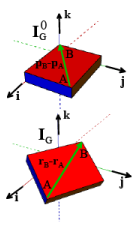Suppose the body is then rotated by a tensor R.

The mass moment of inertia after rotation is given by

IG = RI0GRT

Example: The prism shown in the figure is rotated by 45 degrees about the k axis.   Calculate the mass moment of inertia after the rotation Start by calculating the rotation (use the formulas from 6.2.1)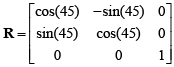We know the inertia tensor of the prism before it is rotated is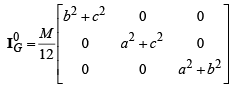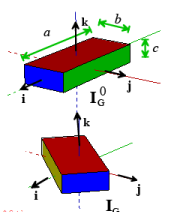We can use Mupad to do the tedious matrix multiplications

[restart () :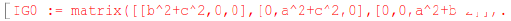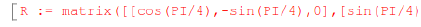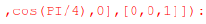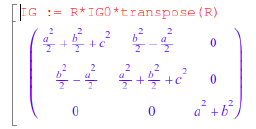Note that the inertia tensor is no longer diagonal.

Rotation formula for 2D motion: Fortunately, 2D is simple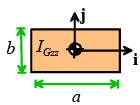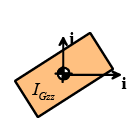Rotating a 2D object about the k axis does not change IGzz

Proof of the rotation formula: Consider a system of N particles.  Suppose that before rotation, the particles are at positions di0 relative to the COM. The initial inertia tensor is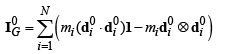Now rotate the system, so the particle s move to new positions di = Rdi.  The new inertia tensor is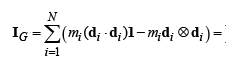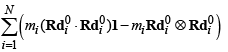It is easy to show (just write out the matrix products)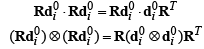which shows that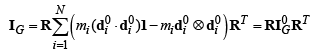Offer running on EduRev: Apply code STAYHOME200 to get INR 200 off on our premium plan EduRev Infinity!

## Introduction to Dynamics and Vibrations- Notes, Videos, MCQs

20 videos|53 docs

,

,

,

,

,

,

,

,

,

,

,

,

,

,

,

,

,

,

,

,

,

,

,

,

;